# csaps

Cubic smoothing spline

## Syntax

``pp = csaps(x,y)``
``pp = csaps(x,y,p)``
``pp = csaps(x,y,p,[],w)``
``values = csaps(x,y,p,xx)``
``values = csaps(x,y,p,xx,w)``
``[___] = csaps({x1,...,xm},y,___)``
``[___,P] = csaps(___)``

## Description

``` NoteFor a simpler but less flexible method to generate smoothing splines, try the Curve Fitter app or the `fit` function. `pp = csaps(x,y)` returns the cubic smoothing spline interpolation to the given data `(x,y)` in ppform. The value of spline f at data site `x(j)` approximates the data value `y(:,j)` for ```j = 1:length(x)```. The smoothing spline f minimizesHere, n is the number of entries of `x` and the integral is over the smallest interval containing all the entries of `x`. yj and xj refer to the jth entries of `y` and `x`, respectively. D2f denotes the second derivative of the function f.The default values for the error measure weights wj are 1. The default value for the piecewise constant weight function λ in the roughness measure is the constant function 1. By default, `csaps` chooses a value for the smoothing parameter p based on the given data sites `x`.To evaluate a smoothing spline outside its basic interval, you must first extrapolate it. Use the command `pp = fnxtr(pp)` to ensure that the second derivative is zero outside the interval spanned by the data sites.```

example

````pp = csaps(x,y,p)` specifies the smoothing parameter `p`. You can also supply the roughness measure weights λ by providing `p` as a vector whose first entry is `p` and `i`th entry is the value of λ on the interval `(x(i-1),x(i))`. ```

example

````pp = csaps(x,y,p,[],w)` also specifies the weights `w` in the error measure.```

example

````values = csaps(x,y,p,xx)` uses the smoothing parameter `p` and returns the values of the smoothing spline evaluated at the points `xx`. This syntax is the same as `fnval(csaps(x,y,p),xx)`. ```
````values = csaps(x,y,p,xx,w)` uses the smoothing parameter `p` and the error measure weights `w`, and returns the values of the smoothing spline evaluated at the points `xx`. This syntax is the same as `fnval(csaps(x,y,p,[],w),xx)````
````[___] = csaps({x1,...,xm},y,___)` provides the ppform of an `m`-variate tensor-product smoothing spline to data on the rectangular grid described by `{x1,...,xm}`. You can use this syntax with any of the arguments in the previous syntaxes. ```

example

````[___,P] = csaps(___)` also returns the value of the smoothing parameter used in the final spline result whether or not you specify `p`. This syntax is useful for experimentation in which you can start with `[pp,P] = csaps(x,y)` and obtain a reasonable first guess for `p`.```

## Examples

collapse all

Fit smoothing splines using the `csaps` function with different values for the smoothing parameter `p`. Use values of `p` between the extremes of 0 and 1 to see how they affect the shape and closeness of the fitted spline.

`[x, y] = titanium();`

When `p = 0`, `s0` is the least-squares straight line fit to the data. When `p = 1`, `s1` is the variational, or natural, cubic spline interpolant.

For `0 < p < 1`, `sp` is a smoothing spline that is a trade-off between the two extremes: smoother than the interpolant `s1` and closer to the data than the straight line `s0`.

```p = 0.00009; s0 = csaps(x,y,0); sp = csaps(x,y,p); s1 = csaps(x,y,1);```
```figure fnplt(s0); hold on fnplt(sp); fnplt(s1); plot(x,y,'ko'); hold off title('Smoothing splines with different values for p'); legend('p = 0', ['p = ' num2str( p )], 'p = 1', 'Location', 'northwest')```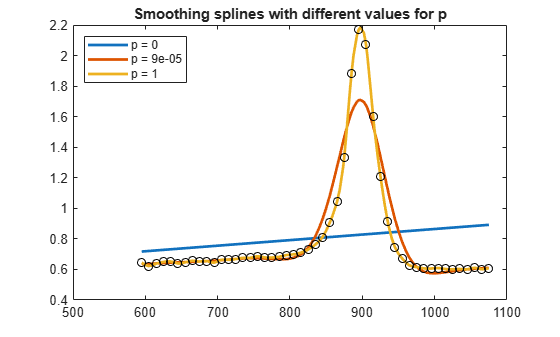Adjust the smoothing parameter, error measure weights, and roughness measure weights.

Create a sine curve with noise.

`x = linspace(0,2*pi,21); y = sin(x)+(rand(1,21)-.5)*.3;`

Fit a smoothing spline to the data. Specify the smoothing parameter `p = 0.4` and error measure weights `w` that vary across the data.

`pp = csaps(x,y,0.4,[],[ones(1,10),repmat(5,1,10), 0]);`

The function returns a smooth fit to the noisy data that is much closer to the data in the right half because of the much larger error measure weight there. Note that the error weighting of zero for the last data point excludes this point from the fit.

Now fit a smoothing spline using the same data, smoothing parameter and error measure weights, but with adjusted roughness measure weights.

```pp1 = csaps(x,y, [.4,ones(1,10),repmat(.2,1,10)], [], ... [ones(1,10), repmat(5,1,10), 0]);```

The roughness measure weight is only 0.2 in the right half of the interval. Correspondingly, the fit is rougher but closer on the right side of the data (except for the last data point, which is ignored).

Plot both fits for comparison.

```figure hold on fnplt(pp, 'b'); fnplt(pp1,'r--') plot(x,y,'ok') hold off ylim([-1.5 1.5]) title(['Cubic smoothing spline, with right half treated ',... 'differently']) legend('Larger error weight', 'Larger error and smaller roughness weight')```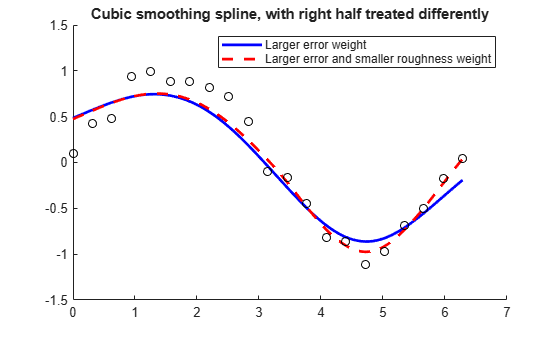Fit a smoothing spline to bivariate data generated by the `peaks` function with added uniform noise. Use `csaps` to obtain the new, smoothed data points and the smoothing parameters `csaps` determines for the fit.

Create the grid. For this example, the grid is a 51-by-61 uniform grid.

```x = {linspace(-2,3,51),linspace(-3,3,61)}; [xx,yy] = ndgrid(x{1},x{2}); ```

Generate the noisy data using the `peaks` function and random numbers in the interval $\left[-\frac{1}{2},\frac{1}{2}\right]$.

```y = peaks(xx, yy); noisy = y + (rand(size(y)) - 0.5); figure surf(xx,yy,noisy) axis off```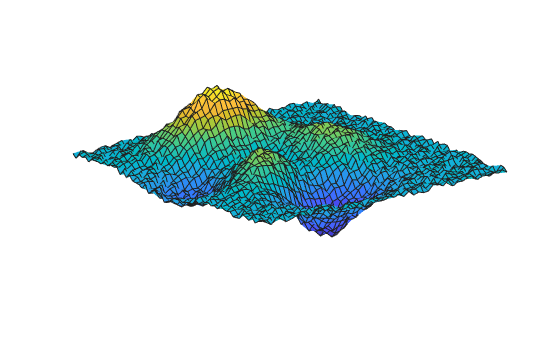Fit the data. Use `csaps` to obtain the smoothed data values evaluated over the grid `x` and the default smoothing parameter used in the fit.

`[sval,p] = csaps(x,noisy,[],x);`

The plot of the fit shows that some roughness remains. Note that you must transpose the array `sval`.

```figure surf(x{1},x{2},sval.') axis off```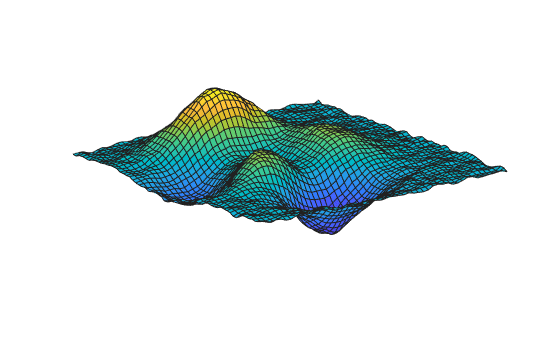For a somewhat smoother approximation, specify a value for `p` that is slightly smaller than the `csaps` default value.

```ssval = csaps(x,noisy,.996,x); figure surf(x{1},x{2},ssval.') axis off```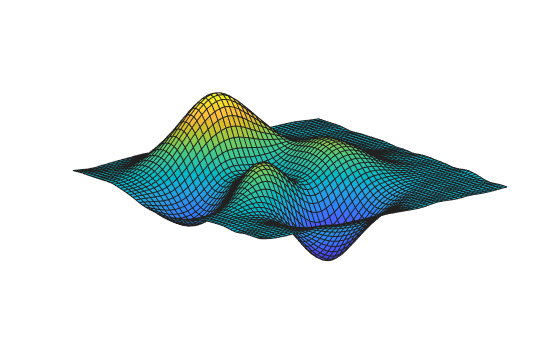## Input Arguments

collapse all

Data sites of data values `y` to be fit, specified as a vector or as a cell array for multivariate data. Spline f is created with knots at each data site `x` such that f(`x(j)`) = `y(:,j)` for all values of `j`.

For multivariate, gridded data, you can specify `x` as a cell array that specifies the data site in each variable dimension: f(`x1(i),x2(j),...xn(k)`) = `y(:,i,j,...,k)`.

Data Types: `single` | `double`

Data values to fit during creation of the spline, specified as a vector, matrix, or array. Data values `y(:,j)` can be scalars, matrices, or n-dimensional arrays. Data values given at the same data site `x` are averaged.

Data Types: `single` | `double`

Smoothing parameter, specified as a scalar value between 0 and 1 or as a cell array of values for multivariate data. You can also specify values for the roughness measure weights λ by providing `p` as a vector. To provide roughness measure weights for multivariate data, use a cell array of vectors. If you provide an empty array, the function chooses a default value for `p` based on the data sites `x` and the default value of `1` for the roughness measure weight λ.

The smoothing parameter determines the relative weight to place on the contradictory demands of having f be smooth or having f be close to the data. For `p` = 0, f is the least-squares straight-line fit to the data. For `p` = 1, f is the variational, or natural, cubic spline interpolant. As `p` moves from 0 to 1, the smoothing spline changes from one extreme to the other.

The favorable range for `p` is often near 1/(1 + h3/6), where h is the average spacing of the data sites. The function chooses a default value for `p` within this range. For uniformly spaced data, you can expect a close fit with p = 1(1 + h3/60) and some satisfactory smoothing with p = 1/(1 + h3/0.6). You can input `p > 1`, but this choice leads to a smoothing spline even rougher than the variational cubic spline interpolant.

If the input `p` is negative or empty, then the function uses the default value for `p`.

You can specify the roughness measure weights λ alongside the smoothing parameter by providing `p` as a vector. This vector must be the same size as `x`, with the `i`th entry the value of λ on the interval `(x(i-1)...x(i))`, for `i = 2:length(x)`. The first entry of the input vector `p` is the desired value of the smoothness parameter `p`. By providing roughness measure weights, you can make the resulting smoothing spline smoother (with larger weight values) or closer to the data (with smaller weight values) in different parts of the interval. Roughness measure weights must be nonnegative.

If you have difficulty choosing `p` but have some feeling for the size of the noise in `y`, consider using `spaps(x,y,tol)` instead. This function chooses `p` such that the roughness measure is as small as possible, subject to the condition that the error measure does not exceed `tol`. In this case, the error measure usually equals the specified value for `tol`.

Data Types: `single` | `double`

Error measure weights w in the error measure, specified as a vector of nonnegative entries of the same size as `x`.

The default value for the weight vector `w` in the error measure is `ones(size(x))`.

Evaluation points over which the spline is evaluated, specified as a vector or as a cell array of vectors for multivariate data. Spline evaluation is performed using `fnval`.

Data Types: `single` | `double`

## Output Arguments

collapse all

Spline in ppform, returned as a structure with these fields.

Form of the spline, returned as `pp`. `pp` indicates that the spline is given in piecewise polynomial form.

Knot positions of the spline, returned as a vector or as a cell array of vectors for multivariate data. Vectors contain strictly increasing elements that represent the start and end of each of the intervals over which the polynomial pieces are defined.

Coefficients of polynomials for each piece, returned as a matrix or as an array for multivariate data.

Number of polynomial pieces describing the spline, returned as a scalar or as a vector of numbers of pieces in each variable for multivariate data.

Order of the polynomial function describing each polynomial piece of the spline, returned as a scalar or as a vector containing the order in each variable for multivariate data.

Dimensionality of the target function, returned as a scalar.

Evaluated spline, returned as a vector or as a matrix or array for multivariate data. The spline is evaluated at the given evaluation points `xx`.

Smoothing parameter used to calculate the spline, returned as a scalar or as a cell array of scalar values for multivariate data. `P` is between `0` and `1`.

## Algorithms

`csaps` is an implementation of the Fortran routine `SMOOTH` from PGS.

The calculation of the smoothing spline requires solving a linear system whose coefficient matrix has the form `p*A + (1-p)*B`, with the matrices `A` and `B` depending on the data sites `x`. The default value of `p` makes `p*trace(A)` equal `(1-p)*trace(B)`.

## Version History

Introduced before R2006a